Anzeige

# gauss

11. Mar 2013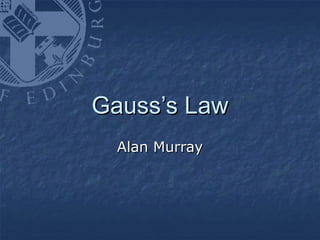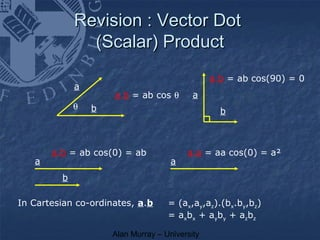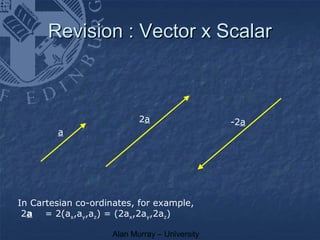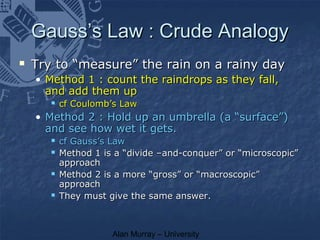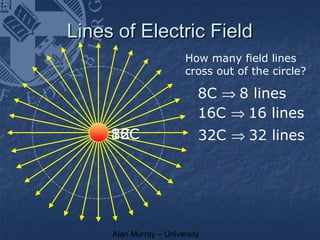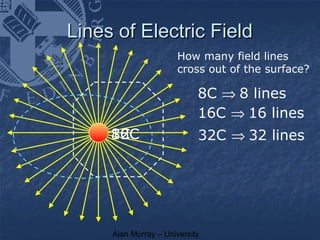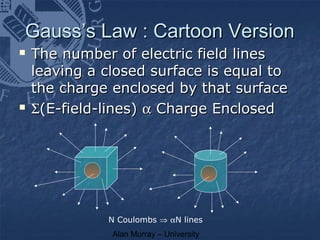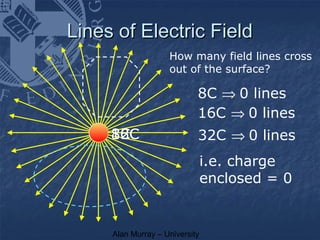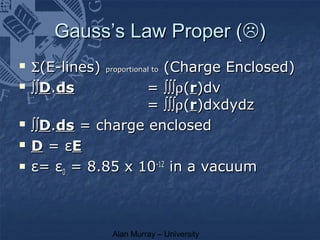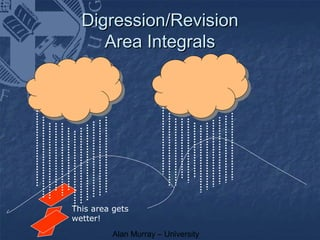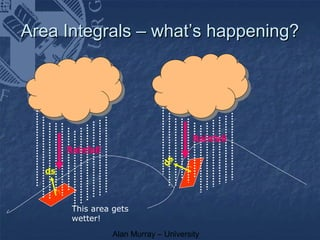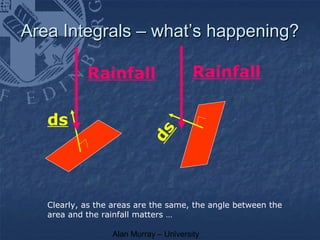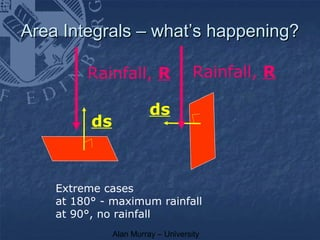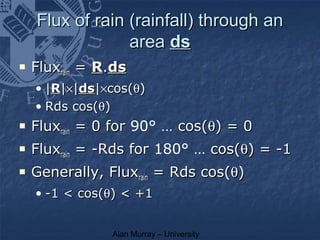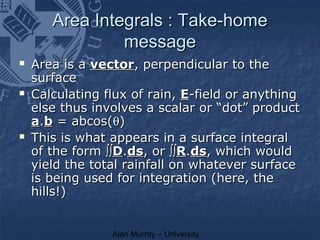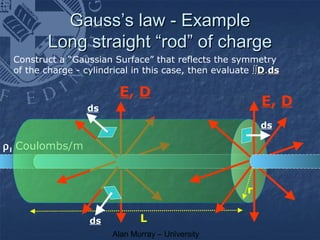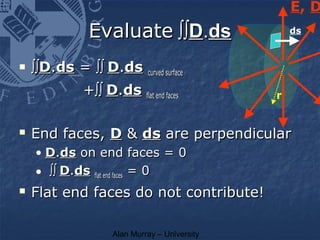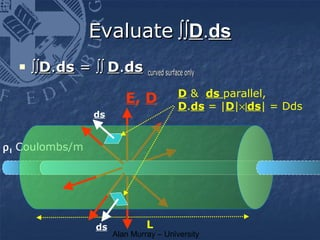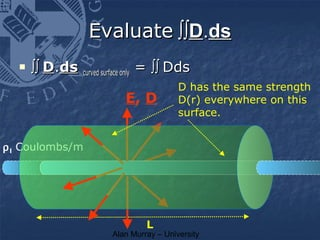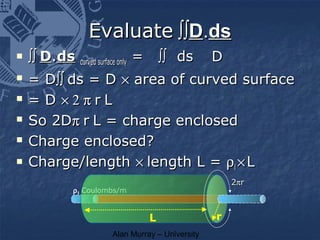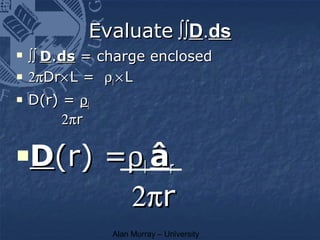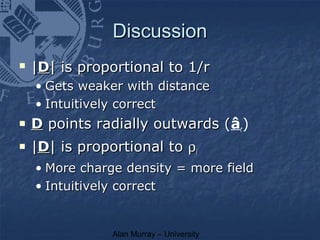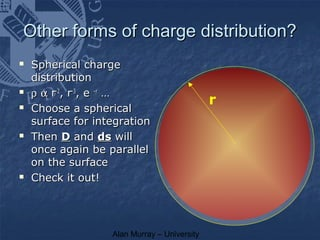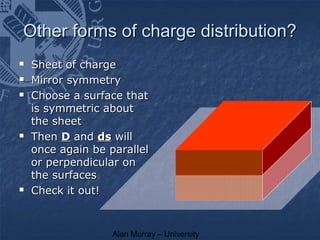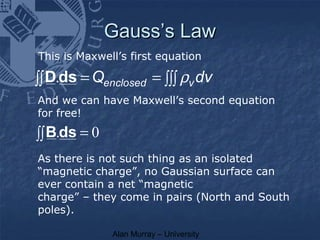1 von 25
Anzeige

### gauss

1. Gauss’s Law Alan Murray
2. Revision : Vector Dot (Scalar) Product a.b = ab cos(90) = 0 a a.b = ab cos θ a θ b b a.b = ab cos(0) = ab a.a = aa cos(0) = a² a a b In Cartesian co-ordinates, a.b = (ax,ay,az).(bx.by,bz) = axbx + ayby + azbz Alan Murray – University
3. Revision : Vector x Scalar 2a -2a a In Cartesian co-ordinates, for example, 2a = 2(ax,ay,az) = (2ax,2ay,2az) Alan Murray – University
4. Gauss’s Law : Crude Analogy  Try to “measure” the rain on a rainy day • Method 1 : count the raindrops as they fall, and add them up  cf Coulomb’s Law • Method 2 : Hold up an umbrella (a “surface”) and see how wet it gets.  cf Gauss’s Law  Method 1 is a “divide –and-conquer” or “microscopic” approach  Method 2 is a more “gross” or “macroscopic” approach  They must give the same answer. Alan Murray – University
5. Lines of Electric Field How many field lines cross out of the circle? 8C ⇒ 8 lines 16C ⇒ 16 lines 32C 16C 8C 32C ⇒ 32 lines Alan Murray – University
6. Lines of Electric Field How many field lines cross out of the surface? 8C ⇒ 8 lines 16C ⇒ 16 lines 32C 16C 8C 32C ⇒ 32 lines Alan Murray – University
7. Gauss’s Law : Cartoon Version  The number of electric field lines leaving a closed surface is equal to the charge enclosed by that surface  Σ(E-field-lines) α Charge Enclosed N Coulombs ⇒ αN lines Alan Murray – University
8. Lines of Electric Field How many field lines cross out of the surface? 8C ⇒ 0 lines 16C ⇒ 0 lines 32C 16C 8C 32C ⇒ 0 lines i.e. charge enclosed = 0 Alan Murray – University
9. Gauss’s Law Proper ()  Σ(E-lines) (Charge Enclosed) proportional to  ∫∫D.ds = ∫∫∫ρ(r)dv = ∫∫∫ρ(r)dxdydz  ∫∫D.ds = charge enclosed  D = εE  ε= ε0 = 8.85 x 10-12 in a vacuum Alan Murray – University
10. Digression/Revision Area Integrals This area gets wetter! Alan Murray – University
11. Area Integrals – what’s happening? Rainfall Rainfall ds ds This area gets wetter! Alan Murray – University
12. Area Integrals – what’s happening? Rainfall Rainfall ds ds Clearly, as the areas are the same, the angle between the area and the rainfall matters … Alan Murray – University
13. Area Integrals – what’s happening? Rainfall, R Rainfall, R ds ds Extreme cases at 180° - maximum rainfall at 90°, no rainfall Alan Murray – University
14. Flux of rain (rainfall) through an area ds  Fluxrain = R.ds • |R|×|ds|×cos(θ) • Rds cos(θ)  Fluxrain = 0 for 90° … cos(θ) = 0  Fluxrain = -Rds for 180° … cos(θ) = -1  Generally, Fluxrain = Rds cos(θ) • -1 < cos(θ) < +1 Alan Murray – University
15. Area Integrals : Take-home message  Area is a vector, perpendicular to the surface  Calculating flux of rain, E-field or anything else thus involves a scalar or “dot” product a.b = abcos(θ)  This is what appears in a surface integral of the form ∫∫D.ds, or ∫∫R.ds, which would yield the total rainfall on whatever surface is being used for integration (here, the hills!) Alan Murray – University
16. Gauss’s law - Example Long straight “rod” of charge Construct a “Gaussian Surface” that reflects the symmetry of the charge - cylindrical in this case, then evaluate ∫∫D.ds E, D ds E, D ds ρ l Coulombs/m r ds L Alan Murray – University
17. E, D Evaluate ∫∫D.ds ds  ∫∫D.ds = ∫∫ D.ds curved surface +∫∫ D.ds flat end faces r  End faces, D & ds are perpendicular • D.ds on end faces = 0 • ∫∫ D.ds flat end faces = 0  Flat end faces do not contribute! Alan Murray – University
18. Evaluate ∫∫D.ds  ∫∫D.ds = ∫∫ D.ds curved surface only D & ds parallel, E, D D.ds = |D|×|ds| = Dds ds ρ l Coulombs/m ds L Alan Murray – University
19. Evaluate ∫∫D.ds  ∫∫ D.ds curved surface only = ∫∫ Dds D has the same strength E, D D(r) everywhere on this surface. ρ l Coulombs/m L Alan Murray – University
20. Evaluate ∫∫D.ds  ∫∫ D.ds curved surface only = ∫∫ ds D  = D∫∫ ds = D × area of curved surface  = D ×2πrL  So 2Dπ r L = charge enclosed  Charge enclosed?  Charge/length × length L = ρl × L 2πr ρ l Coulombs/m L r Alan Murray – University
21. Evaluate ∫∫D.ds  ∫∫ D.ds = charge enclosed  2πDr× L = ρl × L  D(r) = ρl 2πr  D(r) =ρl âr 2πr Alan Murray – University
22. Discussion  |D| is proportional to 1/r • Gets weaker with distance • Intuitively correct  D points radially outwards (âr) (  |D| is proportional to ρl • More charge density = more field • Intuitively correct Alan Murray – University
23. Other forms of charge distribution?  Spherical charge distribution  ρ α r-2, r-3, e –r … r  Choose a spherical surface for integration  Then D and ds will once again be parallel on the surface  Check it out! Alan Murray – University
24. Other forms of charge distribution?  Sheet of charge  Mirror symmetry  Choose a surface that is symmetric about the sheet  Then D and ds will once again be parallel ` ` or perpendicular on the surfaces  Check it out! Alan Murray – University
25. Gauss’s Law This is Maxwell’s first equation ∫∫ D.ds = Qenclosed = ∫∫∫ ρv dv And we can have Maxwell’s second equation for free! ∫∫ B.ds = 0 As there is not such thing as an isolated “magnetic charge”, no Gaussian surface can ever contain a net “magnetic charge” – they come in pairs (North and South poles). Alan Murray – University

### Hinweis der Redaktion

1. Let&apos;s revise some basics first. Given two vectors, a and b, we can do two different forms of multiplication. The scalar, or &quot;dot&quot; product is the easier and the first we will meet. The scalar product of two vectors is the related to the PROJECTION of one on to the other. You can think of this as the shadow cast by one on the other if you like. Thus the scalar product is zero if they are perpendicular, maximum if they are parallel and in betwen otherwise. Formally, it&apos;s given by the formulae shown in terms of angle and magnitudes, or in cartesian co-ordinates as shown here.
2. and just as a sanity-check, here&apos;s what happens when we multiply a vetor by a scalar - it simply changes length (and possibly direction)
3. Let’s do this in cartoon form first. The ethos of Gauss is that we look, not at the number of lines of field that emanate from a point charge and then add them up (or integrate them) but that we effectively count the number of field lines leaving a closed surface (actually, “we find the total electric flux” … more later). What we will then find is that if we choose our closed surface carefully, the maths becomes almost trivial. I know that the prospect of surface integrals is causing you a deep sinking feeling, but bear with me – they’re OK and only very simple examples will come your way in this course! Let’s stick to 2D for now and adopt a drawing scheme whereby each coulomb of charge is represented by (or is viewed as being able to generate) 1 electric field line (this is 100% arbitrary!). We’ll choose a circular “surface” with malice aforethought! Then, 8C leads to 8 lines of E-field poking through the surface. 16C leads to 16 E-lines and 32C to 32 E-lines … and so on. The number of electric field lines leaving a closed surface is proportional to the charge enclosed by the surface. If we re-phrase this as “The total electric flux leaving a closed surface is proportional to the charge enclosed by the surface”, then we have actually stated Gauss’s law, and thus Maxwell’s first equation!
4. And the shape of the surface does not matter
5. And, happily, all of this is true in 3D as well.
6. It does matter, however, if the surface does not enclose any charge
7. So - biting the bullet, here is Gauss properly. D is just E multiplied by the dielectric constant ε, which is ε 0 = 8.85 x 10 -12 for a vacuum and more for materials with some dielectric properties. The “charge enclosed” now becomes a volume integral of charge/volume in a closed surface and the “number of E -field lines” becomes the integral of E (actually D ) over the same closed surface. What’s the dot product, D . E , all about, though?
8. Flux density D and field E have a direction - and here&apos;s why. Look at the water-flux example once more. The same rainfall has dramatically different effects depending upon its direction. Look at the rectangular “umbrellas”. So - the direction, or orientation, is at least as important as the area. A huge umbrella held at 90° to the rainfall won&apos;t keep you very dry. Mathematically, this means that when we talk about an element of area (perversely, usually called ds ... &quot;s&quot; standing for “surface”) we actually have to make it a vector and give it direction - chosen to be PERPENDICULAR to the surface. With this convention, we call is ds. Get used to this - we will see it again.
9. And here they are in close-up.
10. Here is the same thing in simple maths – the dot product automatically take account of the “foreshortening” effect that makes the same area, in the same flux of rain, get either wetter or not, depending upon it’s angle to the flux.
11. Self-explanatory …
12. So – we’ve suddenly got two of Maxwell’s equations on our hands – Gauss’s Law for electric fields and then the same for magnetic fields, where the fact that magnetic poles always hunt in pairs (N and S poles) means that a (Gaussian) surfaceful of them always contains an equal number of Norths and Souths.
Anzeige